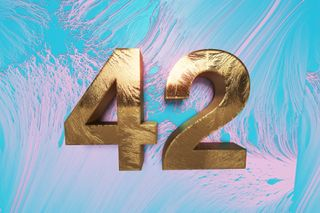# Two Mathematicians Just Solved a Decades-Old Math Riddle — and Possibly the Meaning of LifeIn Douglas Adams' sci-fi series "The Hitchhiker's Guide to the Galaxy," a pair of programmers task the galaxy's largest supercomputer with answering the ultimate question of the meaning of life, the universe and everything. After 7.5 million years of processing, the computer reaches an answer: 42. Only then do the programmers realize that nobody knew the question the program was meant to answer.

Now, in this week's most satisfying example of life reflecting art, a pair of mathematicians have used a global network of 500,000 computers to solve a centuries-old math puzzle that just happens to involve that most crucial number: 42.

The question, which goes back to at least 1955 and may have been pondered by Greek thinkers as early as the third century AD, asks, "How can you express every number between 1 and 100 as the sum of three cubes?" Or, put algebraically, how do you solve x^3 + y^3 + z^3 = k, where k equals any whole number from 1 to 100?

This deceptively simple stumper is known as a Diophantine equation, named for the ancient mathematician Diophantus of Alexandria, who proposed a similar set of problems about 1,800 years ago. Modern mathematicians who revisited the puzzle in the 1950s quickly found solutions when k equals many of the smaller numbers, but a few particularly stubborn integers soon emerged. The two trickiest numbers, which still had outstanding solutions by the beginning of 2019, were 33 and — you guessed it — 42.

In April, mathematician Andrew Booker, of the University of Bristol in England, knocked 33 off the list. Using a computer algorithm to look for solutions to the Diophantine equation with x, y and z values that included every number between positive and negative 99 quadrillion, Booker found the solution to 33 after several weeks of computing time. (As you can see, the answer is super, super long.)Here's 33 expressed as the sum of three cubes. It only took one of the world's smartest computers to solve. (Image credit: Numberphile/ University of Bristol)

Still, this exhaustive search turned up no solutions for 42, suggesting that, if there was an answer, some of the integers must be greater than 99 quadrillion. Calculating values that large would take an insane amount of computing power; so, for his next attempt, Booker enlisted the help of Massachusetts Institute of Technology mathematician Andrew Sutherland, who helped Booker book some time with a worldwide computer network called Charity Engine.

According to a statement from the University of Bristol, this network is a "worldwide computer" that borrows idle computing power from more than 500,000 home PCs around the globe. Using this crowdsourced supercomputer and 1 million hours of processing time, Booker and Sutherland finally found an answer to the Diophantine equation where k equals 42.

And so, without further ado, the question AND answer to the meaning of life, the universe and everything is:

(-80538738812075974)^3 + (80435758145817515)^3 + (12602123297335631)^3 = 42

How does it feel? Glorious? Overwhelming? Like your brain is going to vomit a little? Just be thankful that, unlike in Adams' search for the truth, the entire Earth wasn't destroyed in the process.

Originally published on Live Science.